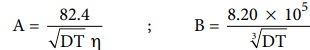Home | | Chemistry 12th Std | Debye - Huckel and Onsager equation

# Debye - Huckel and Onsager equation

The influence of ion-ion interactions on the conductivity of strong electrolytes was studied by Debye and Huckel.

## Debye - Huckel and Onsager equation

We have learnt that at infinite dilution, the interaction between the ions in the electrolyte solution is negligible. Except this condition, electrostatic interaction between the ions alters the properties of the solution from those expected from the free ŌĆō ions value. The influence of ion-ion interactions on the conductivity of strong electrolytes was studied by Debye and Huckel. They considered that each ion is surrounded by an ionic atmosphere of opposite sign, and derived an expression relating the molar conductance of strong electrolytes with the concentration by assuming complete dissociation. Later, the equation was further developed by Onsager. For a uni ŌĆō univalent electrolyte the Debye Huckel and Onsager equation is given below.

╬ø m = ╬ø0m ŌłÆ ( A + B ╬ø0m ) ŌłÜC                          .....(9.12)

Where A and B are the constants which depend only on the nature of the solvent and temperature. The expression for A and B areHere, D is the dielectric constant of the medium, ╬Ę the viscosity of the medium and T the temperature in Kelvin.

Tags : Variation of molar conductivity with concentration | Electro Chemistry | Chemistry , 12th Chemistry : UNIT 9 : Electro Chemistry
Study Material, Lecturing Notes, Assignment, Reference, Wiki description explanation, brief detail
12th Chemistry : UNIT 9 : Electro Chemistry : Debye - Huckel and Onsager equation | Variation of molar conductivity with concentration | Electro Chemistry | Chemistry